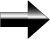The Basics Of Functions

A Function is basically a definition.  It defines one variable in terms or another (or several others).  For example, the function that describes a straight line is f(x) = y = mx + b.  In this function, m is the slope of our straight line, and b is the y-intercept.  Our variables are x and y.

We can see that if we put a number in for x, we will find a value for y.  The value of y depends upon the value of x.  In this function, y is known as a dependent variable, and x is an independent variable.

Main Pre-Algebra Page